AP State Syllabus AP Board 9th Class Physical Science Important Questions Chapter 4 Atoms and Molecules

## AP State Syllabus 9th Class Physical Science Important Questions 4th Lesson Atoms and Molecules

### 9th Class Physical Science 4th Lesson Atoms and Molecules 1 Mark Important Questions and Answers

Question 1.
What is the difference between 2N and N2?
2N means two nitrogen atoms.
N2 means one nitrogen molecule.

Question 2.
Mohan said, “O2 differs from O,”. Do you agree? Justify.
1) Yes, I agree with Mohan’s statement.
2) ‘O’ means single oxygen atom.
‘O2‘ means single oxygen molecule.Question 3.
The atomic number (Z) of an element is 6. Name the element.
Carbon.

Question 4.
Write any one precaution in doing the experiment chromotography.
Make sure that the ink line or mark does not touch the water.

Question 5.
In a class, a teacher asked students to write the molecular formula of Oxygen, Namitha wrote the formula as “O2“, Raju as “O”. Which one is correct?
Namitha is correct.
Molecular formula of oxygen is O2.

### 9th Class Physical Science 4th Lesson Atoms and Molecules 2 Marks Important Questions and Answers

Question 1.
Fill the following table.

 Name Molecular Mass No. of Particles present in molar mass 1. Atomic Oxygen 16 gr 2. Sodium 23 gr 3. Sodium chloride 6.02 × 1023 of Sodium chloride 4. Water 18 gr

 Name Molecular Mass No. of Particles present in molar mass 1. Atomic Oxygen 16 gr 6.02 × 1023 of oxygen atoms 2. Sodium 23 gr 6.02 × 1023 of sodium atoms 3. Sodium chloride 58.5 gr 6.02 × 1023 of Sodium chloride 4. Water 18 gr 6.02 × 1023 of water molecules

Question 2.
Compare the subatomic particles electron, proton and neutron.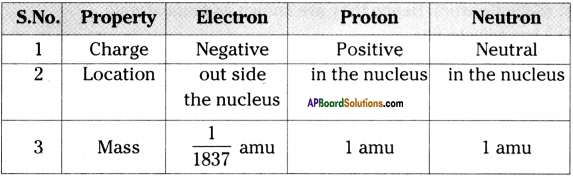Question 3.
What are the materials used in “Conservation of mass” experiment?
Material required for Conservation of mass:
Sodium sulphate, Barium chloride, distilled water, conical flask, spring balance, small test tube, rubber cork, thread, retort stand.

### 9th Class Physical Science 4th Lesson Atoms and Molecules 4 Marks Important Questions and Answers

Question 1.
Fill in the blanks in the table using the given information.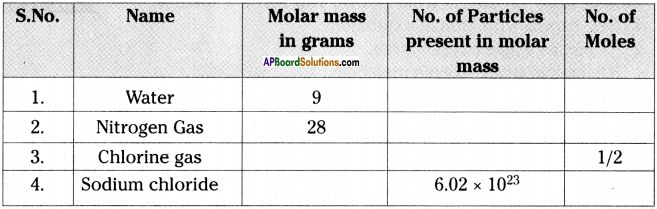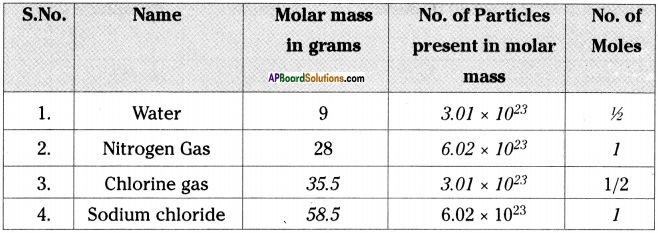Question 2.
Molecular mass of a molecule is the sum of the atomic masses of individual atoms in it.

 Element Atomic number Atomic mass Sodium 11 23 Oxygen 8 16 Hydrogen 1 1 Carbon 6 12

i) Find the molecular mass of Na2CO3.
Molecular mass of Na2CO3 = (23 × 2) + 12 + (16 × 3) = 46 + 12 + 48 = 106

ii) If the molecular weight of a compound is 44. Which is made with carbon and oxygen. What its molecular formula?
Molecular weight of a compound = 44
At. mass of Carbon = 12 Remaining at. mass = 44-12 = 32 = 16 × 2
Hence, 16 × 2 ⇒ (Oxygen atomic mass × 2) ⇒ O2
Molecular formula = CO2

iii) What is the unit of atomic mass?
Unit of atomic mass = amu
atomic mass unit (amu) is defined as precisely $$\frac{1}{12}$$ the mass of an atom of carbon -12.

iv) On the basis of molecular weights of NaOH and H2O, which is heavier?
Molecular weight of NaOH = 23+16 + 1= 40
Molecular weight of H2O = (1 × 2)+ 16 = 2 + 16=18
Hence, NaOH is heavier than H2O.

Question 3.
Complete the table.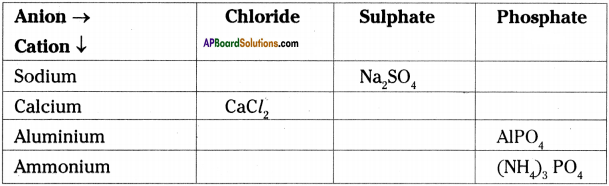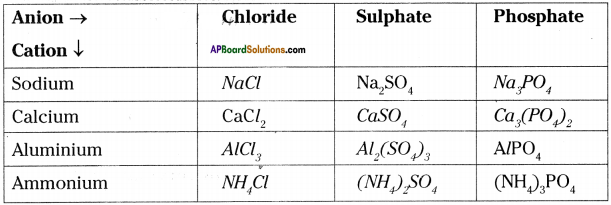### 9th Class Physical Science 4th Lesson Atoms and Molecules Important Questions and Answers

9th Class Physical Science 4th Lesson Atoms and Molecules 1 Mark Important Questions and Answers

Question 1.
How did the element Helium get its name?
Place of discovery of element can also play a role in its naming. The gas which was first discovered in the sun was named Helium. Because, Greek name for sun is helios’.

Question 2.
What is an atom?
An atom is the smallest particle of an element that can participate in chemical reaction and retain all its properties.Question 3.
Is ‘Na’ an element or compound? Why?
‘Na’ is an element, because it was formed by the same atoms of ‘Na’.

Question 4.
Is O2 an element or compound? Why?
O2 is a compound, because O2 is formed by combining two oxygen atoms.

Question 5.
What is an Avogadro number? What is its value?
The number of particles present in one mole of any substance is the Avogadro number (NA).
This is equal to 6.022 × 1023.

Question 6.
Which instrument is used to calculate the atomic mass exactly?
Mass spectrometer.Question 7.
What is atomic mass?
The number of times one atom of given element is heavier than 1/12th part of atomic mass of carbon -12 is called its atomic mass.

Question 8.
How molecules are formed?
A molecule is formed by the combination of different kinds of atoms that are chemically bonded together by attractive forces.

Question 9.
Why is it not possible to see an atom with naked eye?
The size of an atom is so small i.e., less than 1 nm (1 nano metre). So we are unable to see an atom.

Question 10.
What is a chemical formula?
The chemical formula of a compound is a symbolic representation of its composition.

Question 11.
How many atoms are present in.
i) H2S molecule and
ii) PO43- ion?
i) The number of atoms in H2S molecule = 2 + 1=3
ii) The number of atoms in PO43- ion =1 + 4 = 5

9th Class Physical Science 4th Lesson Atoms and Molecules 2 Marks Important Questions and Answers

Question 1.
Who is called as the father of modern chemistry? What are his main contributions?

1. Antoine Lavoisier a French scientist is called as “Father of modern chemistry”.
2. He made many important contributions to chemistry.
3. One of his contributions is law of conservation of mass.

Question 2.
State the following.
a) Law of conservation of mass
b) Law of constant proportions
a) Law of conservation of mass :
Matter is neither created nor destroyed during a chemical reaction.
(or)
The mass of the reactants is equal to the mass of the products of chemical reaction.

b) Law of constant proportions :
A given chemical substance always contains the same elements combined in a fixed proportions by weight.Question 3.
What are the Dalton’s proposals about the nature of matter?
John Dalton proposed the basic theory about the nature of matter. His proposals are :

1. If mass was to be conserved, then all elements must be made up of extremely small particles called atoms.
2. If law of constant proportions is to be followed, the particles of same substance couldn’t be dissimilar.

Question 4.

1. About 2600 years ago, an Indian sage called Kanada, postulated atoms in his Vaishesika sutra”.
2. He proposed that all forms of matter are composed of very small particles known as “Anu”.
3. Each “Anu” may be made up of still smaller particles called “Paramanu”.

Question 5.
What is the use of symbols for elements?

1. We know that chemistry involves a lot of reactions.
2. It will be a waste of time to write the full name of the elements and compounds every time to describe a reaction.
3. To avoid this we use symbols for naming the elements and formulae to represent compounds.

Question 6.
What are the characteristics of a symbol?
1) A symbol can have either one or two letters of English.
Ex : H, He, N, Ne, etc.

2) The first letter of the symbol is always upper case and the second letter is always lower case.
Ex :
1) Al, Cr, Cl, etc. is the right method to represent elements.
2) CL, bE, he, etc. is the wrong method to represent elements.Question 7.
In a reaction, 5.3 g of sodium carbonate reacted with 6 g of ethanoic acid. The products were 2.2 g of carbon dioxide, 0.9 g of water, and 8.2 g of sodium ethanoate. Show that these observations are in agreement with law of conservation of mass.
Sodium carbonate + Ethanoic acid → Sodium ethanoate + Carbon dioxide + Water
The total mass of reactants = 5.3 + 6 = 11.3 g
The total mass of products = 2.2 + 0.9 + 8.2 = 11.3 g
∴ Total mass of reactants = Total mass of products
So these observations are in agreement with law of conservation of mass.

Question 8.
Hydrogen and oxygen combine in the ratio of 1 : 8 by mass to form water. What mass of oxygen gas would be required to react completely with 3 g of hydrogen gas?
According to data 1 g of hydrogen is reacting with 8 g of oxygen.
So amount of oxygen would be required to react completely with 3 g of hydrogen
= 3 x 8 = 24 g

Question 9.
Calculate formula unit weight of ZnO, Na2O, K2CO3 (Given atomic weight of Zn = 654)
Formula unit weight of ZnO = 65 + 16 = 81 u
Formula unit weight of Na2O = 2 × 23 +16 = 62u
Formula unit weight of K2CO3 = 2 × 39 + 12 + 3 × 16 = 138 u

Question 10.
Calculate the number of moles for the following.
i) 52 g of He (finding mole from mass).
ii) 12.044 × 1023 number of He atoms (finding mole from number of particles).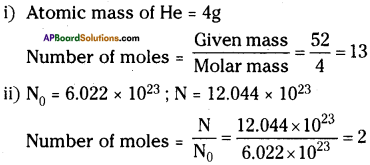Question 11.
If one mole of carbon atom weighs 12 g. What is the mass of 1 atom of carbon?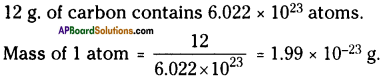Question 12.
When 3 g of carbon is burnt in 8 g of oxygen 11 g of carbon dioxide is produced. What mass of carbon dioxide is formed when 3 g of carbon is burnt in 50 g of oxygen.
3 g of carbon react with 8 g of oxygen to form 11 g of carbon dioxide.
Ratio of carbon and oxygen = 3 : 8
The amount oxygen react with 3g of carbon = 3 × 8 = 24.
So the amount of carbon dioxide formed = 3 + 24 = 27 g.Question 13.
Given the names of the elements present in the following compounds.
a) Quick lime
b) Hydrogen bromide
c) Baking soda
d) Potassium sulphate

 Compound Formula Elements a) Quick lime CaO Calcium, oxygen b) Hydrogen bromide HB2 Hydrogen, bromine c) Baking soda NaHCO3 Sodium, hydrogen, carbon, oxygen d) Potassium sulphate K2SO4 Potassium, sulphur, oxygen

Question 14.
Calculate number of sulphur (Sg) present in 16 g of solid sulphur.
Molecular weight of sulphur = 8 × 32 = 256 g.
256 g of sulphur contains 6.022 × 1023 molecules.
Number of molecules present in 16 g of sulphur = $$\frac{16}{256}$$ × 6.022 × 1023
= 3.77 × 1022 molecules

Question 15.
Anitha wrote the formula for oxygen molecule as 20. Is it correct or not? Why?

1. It is not correct.
2. The formula of oxygen molecule is O2.
3. 2O shows two separate atoms of oxygen.
4. O2 shows molecule of oxygen.

9th Class Physical Science 4th Lesson Atoms and Molecules 4 Marks Important Questions and Answers

Question 1.
How elements got their names? Explain with examples.
I: Sometimes elements are named based on their property.
Ex :
1) The Latin name for water is “hydro”.
So the element that combined with oxygen to give water was named as ‘hydrogen’.

2) The Latin word for acid is ‘oxy’.
Hence the gas that forms acid is ‘oxygen’.

II. Place of discovery of element can also play a role in its naming.
Ex : The gas that was first discovered in the sun (Greek name for the sun is “helios”) was named as ‘helium’.

III. Sometimes the elements were named to honour the scientists.
Ex : Einsteinium, Fermium, Rutherfordium and Mendelevium, etc.Question 2.
Define the following terms.
a) Atomicity
b) Valency
c) Ions
a) Atomicity :
The number of atoms constituting a molecule is known as its atomicity.

Ex :
1) Na is monoatomic
2) O2 is diatomic
3) O3 is triatomic

b) Valency :
Atoms of the elements have power to combine with atoms of other elements. This is known as valency.
Ex :
1) Valency of carbon is 4.
2) Valency of oxygen is 2.
3) Valency of hydrogen is 1.

c) Ions:
1) Ions may be a single charged atoms or a group of atoms that have a net charge on them.
2) A negatively charged ion is called anion and the positive charge ion is cation.
Ex : Na+, Cu+2, S2-, N3-, etc.

Question 3.
Explain the method of writing a formula to a compound using criss – cross method, with the help of an example.
The following steps should be taken while attempting to write a chemical formula using criss – cross method.
Ex : Take sodium carbonate as an example.
1) Write the symbols of atoms or group of atoms side by side, usually the cation first.
NaCO3

2) Write the valency of each atom or group of atoms on the top of its symbol.
Na¹ (CO3

3) Divide the valency number by their highest common factor if any to get the simple ratio.
Na¹ (CO3

4) Interchange the valency and write the numbers to the lower right of the constituents as subscript.
Na2(CO3)1

5) If any constituent receives the number 1, ignore it while writing the formula.
Na2CO3

6) If group of atoms received the number more than 1, enclose it within brackets. Hence the formula for the sodium carbonate is Na2CO3.

Question 4.
Define the terms :
a) molecular mass,
b) formula unit mass,
c) mole and
d) molar mass.
a) Molecular mass :
The molecular mass of a substance is the sum of the atomic masses of all the atoms in a molecule of a substance.

b) Formula unit mass :
The formula unit mass of a substance is a sum of the atomic masses of all atoms in a formula unit of a compound.

c) Mole :
1) One mole of a substance is the amount of the substance which contains as many particles or entities that are equal to the atoms present in exactly 12 grams of C12 isotope.
2) The number of particles present in one mole of any substance has a fixed value of 6.022 × 1023. This number is called Avogadro’s constant (NA).

d) Molar mass :
The mass of 1 mole of a substance which is expressed in grams is called its molar mass.Question 5.
What are the main postulates of Dalton’s atomic theory?
The main postulates of Dalton’s atomic theory are :

1. Matter consists of indivisible particles called atoms.
2. Atoms are neither created nor destroyed in a chemical reaction, but atoms will reorganize.
3. All the atoms of a given element have identical mass and chemical properties.
4. Atoms of different elements have different masses and chemical properties.
5. Compounds are formed when atoms of different elements combine in simple whole number ratios i.e., chemical change is the union or separation of atoms as a whole number.

Question 6.
Draw the pie diagram to understand the atomic mass and explain it.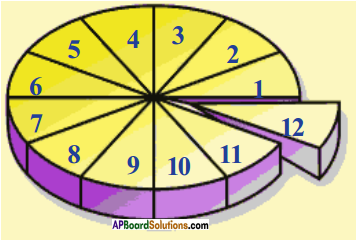Explanation :

1. Let us assume the circle in the diagram represents mass of one carbon – 12.
2. It is divided into 12 equal parts as shown in the figure.
3. Each part represents 1/12th of atomic mass of one carbon -12.

Atomic mass unit :
One atomic mass unit is defined as the mass exactly one-twelfth the atomic mass of carbon – 12 isotope.

Question 7.
Illustrate the concept of mole through a diagram.
Mole :

1. One mole of a substance is the amount of the substance which contains as many particles (atoms, molecules etc.) or entities that are equal to the atoms present in exactly 12 grams of 12C isotope.
2. The number of particles present in one mole of any substance has a fixed value of 6.022 × 1023. This number is called Avogadro’s constant (NA).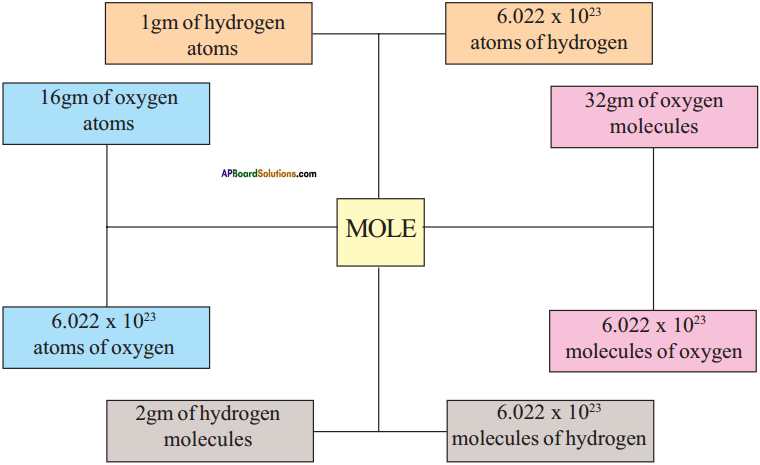Question 8.
How can you appreciate John Dalton for proposing his atomic theory?

• We know, development of science and technology is a combined effort of Roman scientists.
• The zeal to know or discover something new, leads the scientists for their discoveries.
• One of such is the atomic theory proposed by Dalton, based on Lavoisier’s law of conservation of mass and Proust’s law of constant proportions.
• Dalton said atom is indivisible.
• This proposal lead to discover many new things by various scientists and to unveil the complete structure of atom.
• Hence Dalton’s contribution is highly appreciable.

Question 9.
How do you feel after studying the symbols and formulae of different elements and compounds?

• In my earlier class I came to know that chemistry is nowhere but in our kitchen.
• I used to call so many chemicals with their names.
• Now I am able to call them with their symbols and formulae.
• For example 1 can call the common salt as NaCl.
• This thrills me alot, and I am curious to know the formulae of so many common household substances.
• This is very helpful for my higher studies.Question 10.
Describe the experiment conducted by Joseph L. Proust, which lead him to propose law of constant proportions.

• Proust took two samples of copper carbonate, one from nature and another prepared in the lab.
• These two samples are chemically decomposed to find percentage of copper, carbon and oxygen.
• The results obtained are given in the table.
 Weight percentage of Natural sample Synthetic sample Copper 51.35 51.35 Carbon 38.91 38.91 Oxygen 9.74 9.74
• From the above table we observe that the percentage of copper, carbon and oxygen atoms in two samples are same.
• Based on this observation Proust proposed the law of constant proportions as “A given chemical substance always contains the same elements combined in a fixed proportions by weight”.

Question 11.
Write down the formulae of these compounds, using criss – cross method?
i) Sodium oxide
ii) Aluminium chloride
iii) Sodium sulphate
iv) Magnesium hydroxide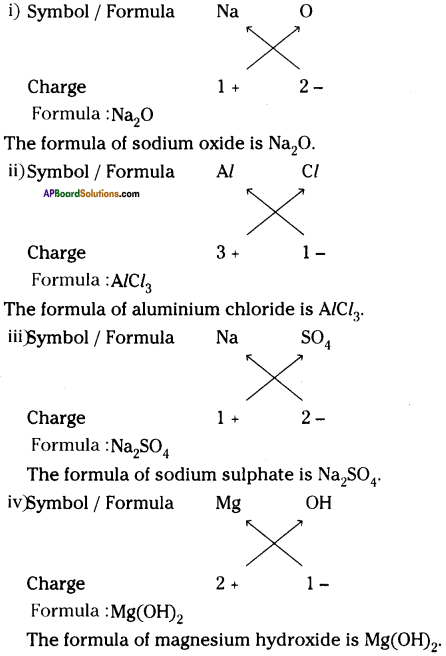Question 12.
Write down the names of compounds represented by the following formulae and also write the anion, cation.
i) Al2(SO4)3
ii) CaCl2
iii) K2SO4
iv) KNO3
v) CaCO3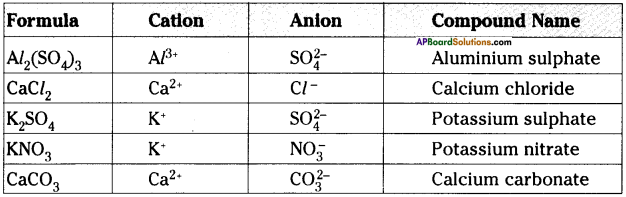Question 13.
What are the rules you have to follow while writing a chemical formula?

1. The valencies or charges on the ion must balance.
2. When a compound consists of metal and non-metal, the name of the symbol of metal is written first, eg : Calcium oxide (CaO)
3. In compounds formed with poly atomic ions, the ion is enclosed in a bracket before writing the number to indicate the ratio.

Question 14.
Calculate the molecular weight H2, O2, Cl2, CO2, CH4, C2H6, C2H4, NH3, CH3OH.
Molecular weight of H2 = 2 × 1 = 2 u
Molecular weight of O2 = 2 × 16 = 32 u
Molecular weight of Cl2 = 2 × 35.5 = 71 U
Molecular weight of CO2 = 12 + 2 × 16 = 44 u
Molecular weight of CH4 = 12 + 4 × 1 = 16 u
Molecular weight of C2H6 = 2 × 12 + 6 × 1= 30 u
Molecular weight of C2H4 = 2 × 12 + 4 × 1= 28 u
Molecular weight of NH3 = 14 + 3 × 1 = 17 u
Molecular weight of CH3OH = 12 + 4 × 1 + 16 = 32 uQuestion 15.
a) What is atomicity?
b) Give examples.
c) Why do elements have different atomicities?